Function Repository Resource:

RoundedPolygon

Represent a polygon with rounded corners

Contributed by: Jan Mangaldan
 ResourceFunction["RoundedPolygon"][{p1,…,pn},r] represents a filled rounded polygon with points pi and rounding radius r. ResourceFunction["RoundedPolygon"][{p1,…,pn},{r1,…,rn}] represents a filled rounded polygon with points pi and corresponding rounding radii ri.

Details

ResourceFunction["RoundedPolygon"] returns a Polygon object, and can be used as a graphics primitive.
ResourceFunction["RoundedPolygon"][poly,rspec], where poly is a Polygon, Rectangle or Triangle object, gives a rounded version of poly.
The rounding radii ri must be nonnegative numbers.
ResourceFunction["RoundedPolygon"] works with 2D and 3D polygons.

Examples

Basic Examples (2)

A triangle with rounded corners:

 In:=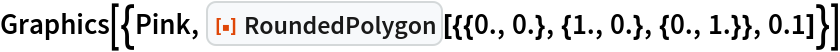Out=A rounded rectangle with different rounding radii for each corner:

 In:=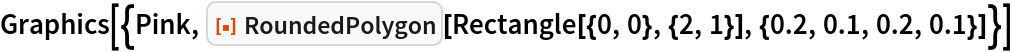Out=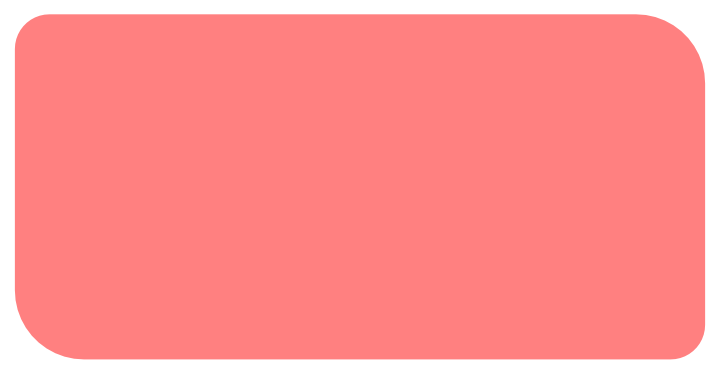Scope (4)

Coordinates for a star-shaped polygon:

 In:=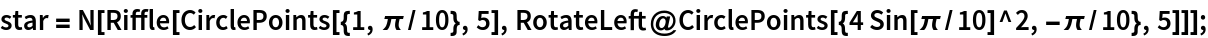Show the original polygon and the rounded version:

 In:=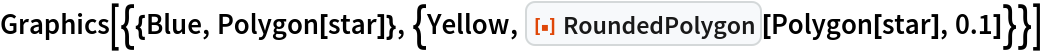Out=Use different rounding radii for each vertex:

 In:=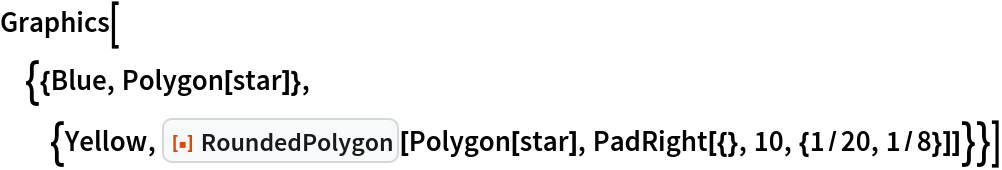Out=A rounded 3D polygon:

 In:=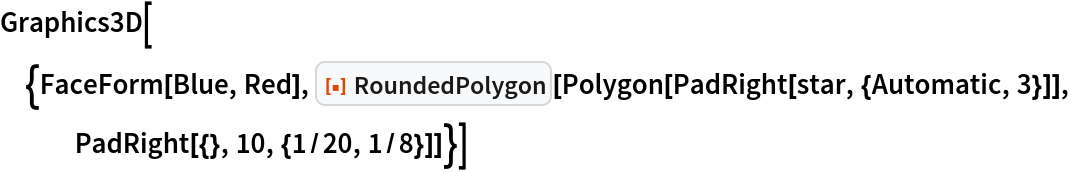Out=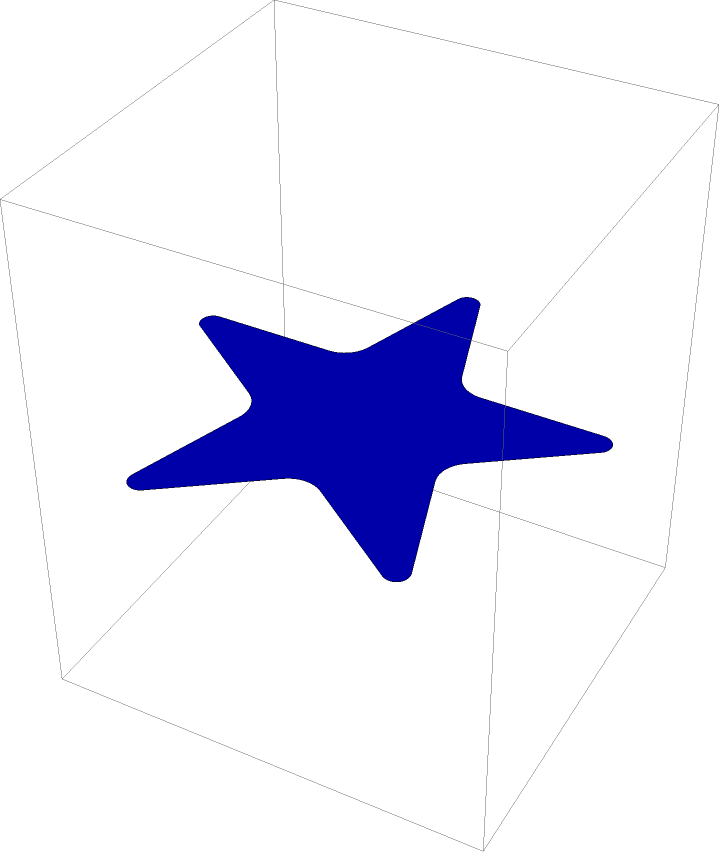Applications (2)

Plot a function over a rounded polygon domain:

 In:=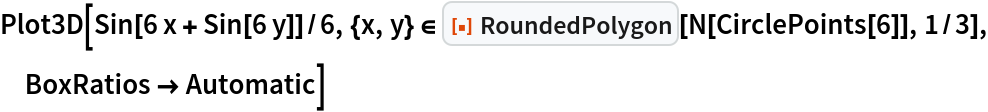Out=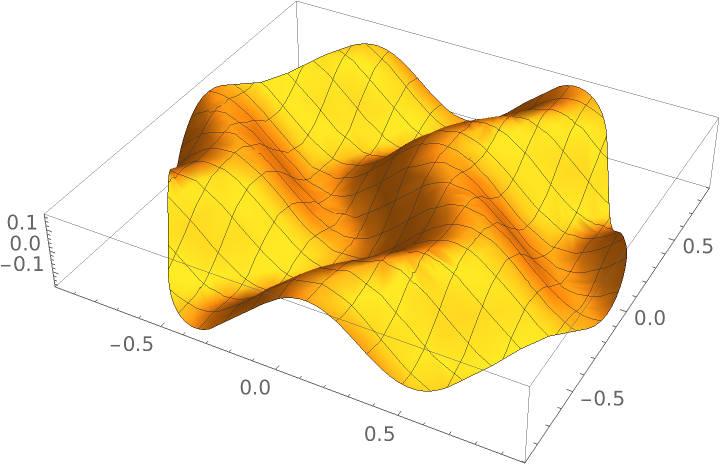A polyhedron with rounded faces:

 In:=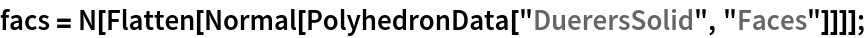In:=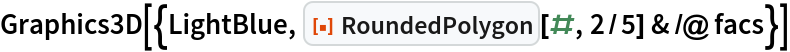Out=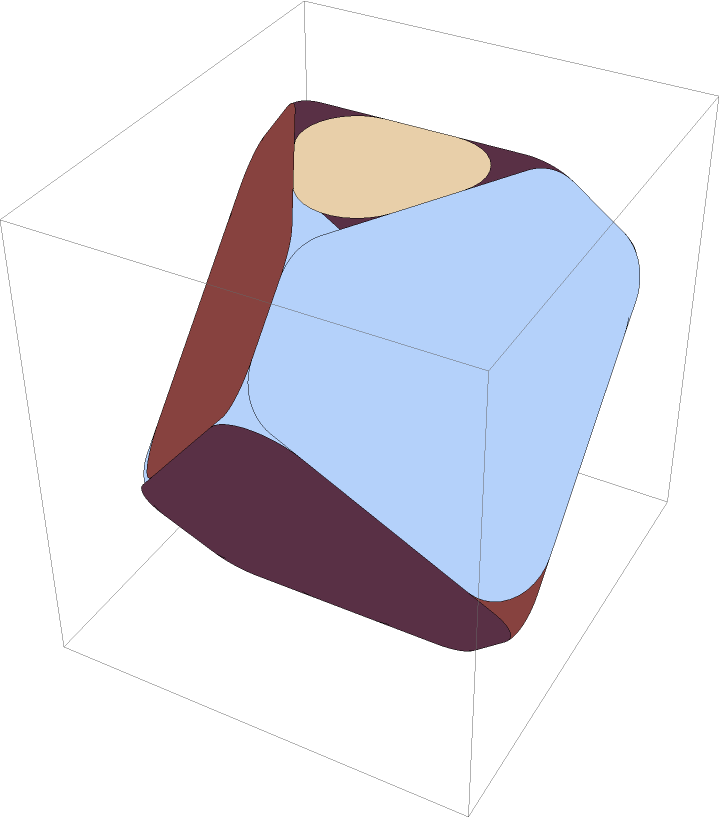Properties and Relations (2)

RoundedPolygon returns a Polygon object:

 In:=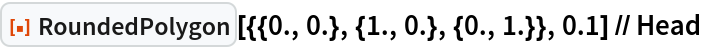Out=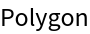Applying RoundedPolygon to a Rectangle is equivalent to setting its RoundingRadius:

 In:=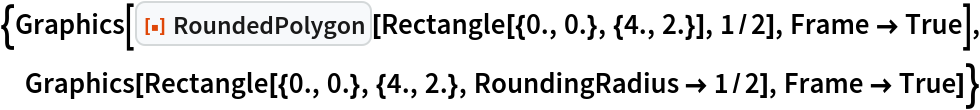Out=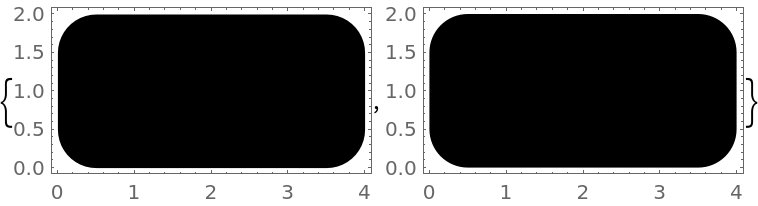Possible Issues (2)

If the rounding radius is too large, RoundedPolygon may give unexpected results:

 In:=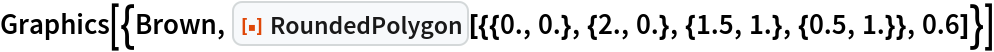Out=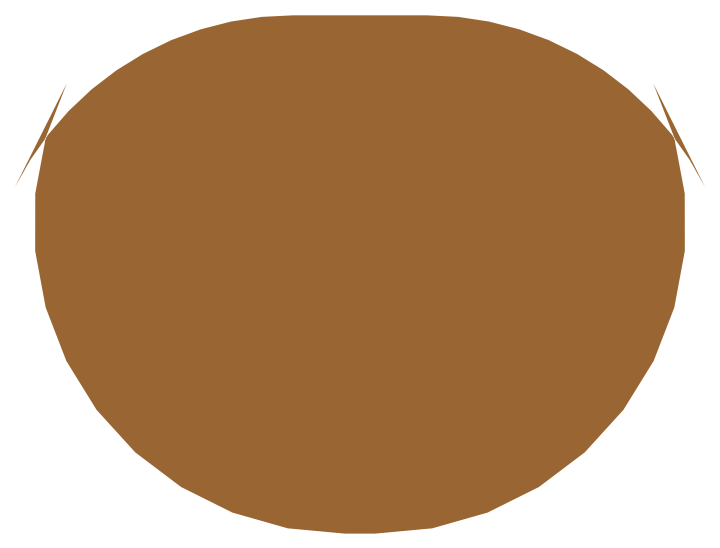In:=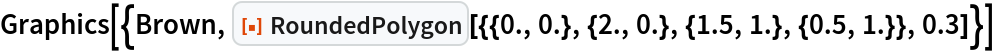Out=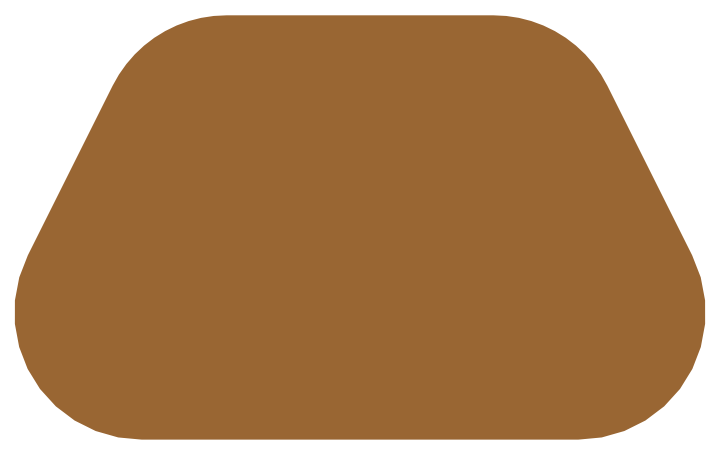Neat Examples (2)

Rounded Voronoi cells:

 In:=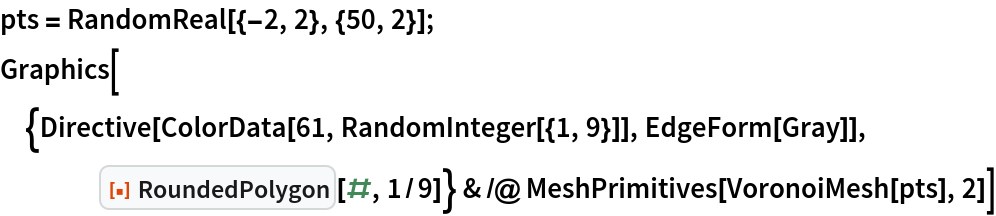Out=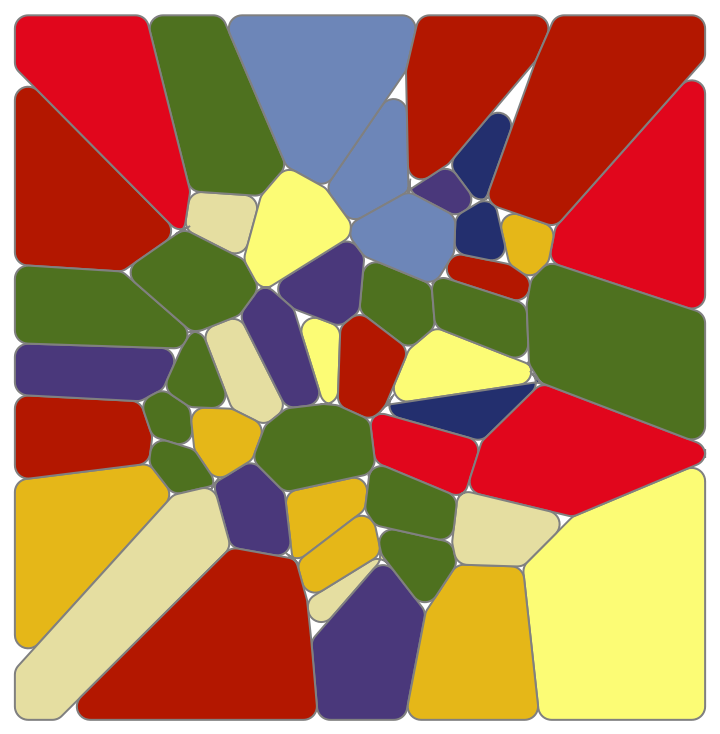Use RoundedPolygon with the resource function PerforatePolygons on a truncated icosahedron:

 In:=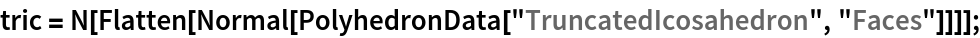In:=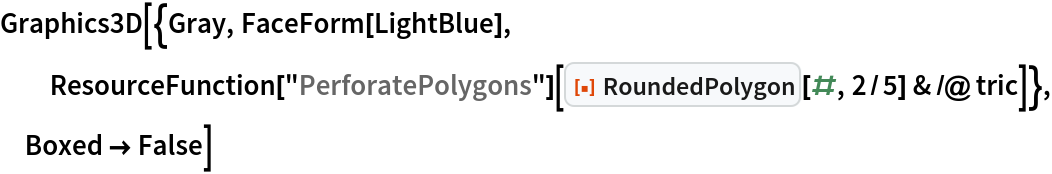Out=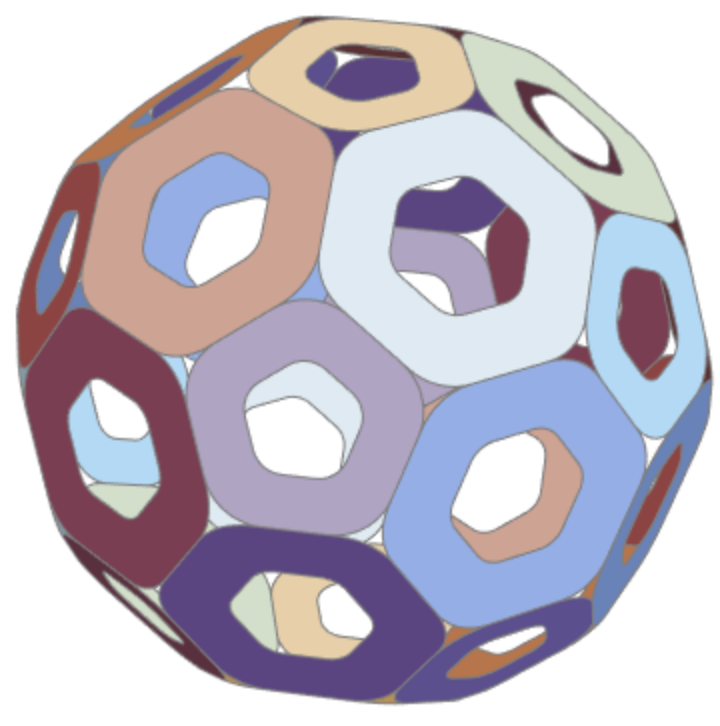Use RoundedPolygon with the resource function OutlinePolygons on a truncated icosahedron:

 In:=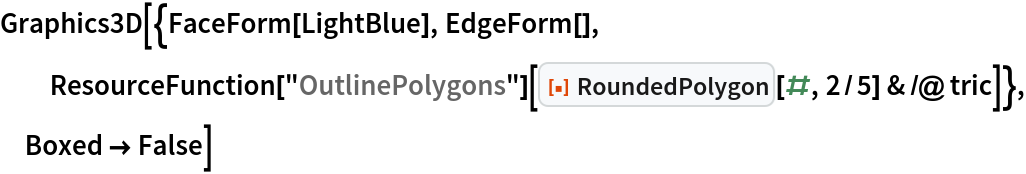Out=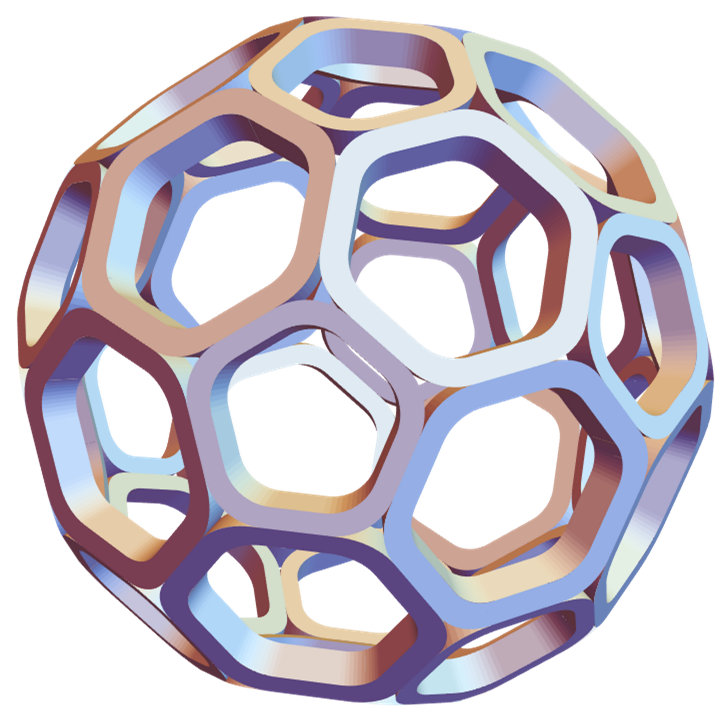Author Notes

Polygons with holes are currently not supported.